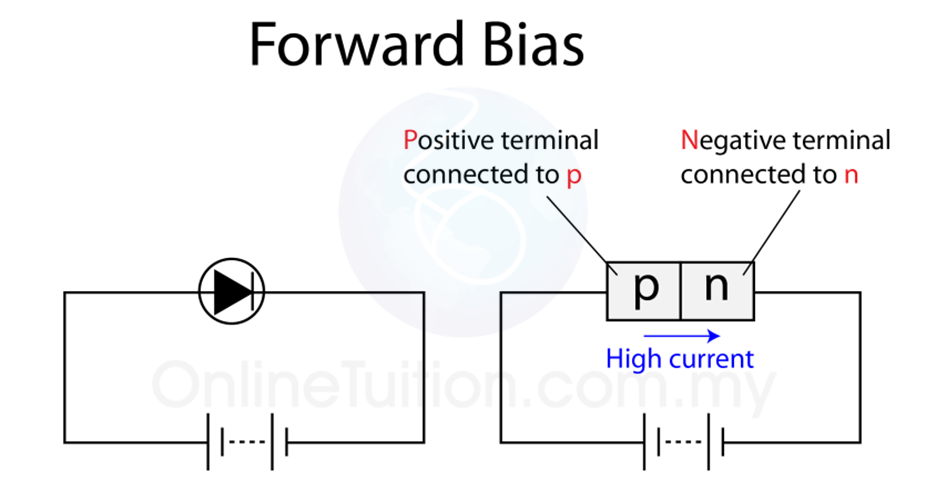Biasing of P-N junction=> P-n junction is a semiconductor that carries current in only one direction

=> applying voltage across the terminals of the diode is called biasing

=> based on the direction of the field applied, there are  two types of biasing

FORWARD BIASING

=> when positive voltage is applied at the P terminal and negative voltage is applied at N terminal of diode, then it is forward biasing. as the name suggests, current flows in this biasing.

characteristics of  diode in forward biasing1. the depletion layer gets thinner because the applied voltage forces the mobile charges to move towards the immobile charge at the junction

2.no current flows when voltage is below barrier potential (0.7v)

3. large current flows when voltage is above barrier potential.

4. current flows due to majority charge carriers

5. offers very low resistance

REVERSE BIASING

=> when the positive voltage is applied across the n-terminal and negative voltage is applied across the p-terminal.

=> current doesnt  flow until very high breakdown voltage is applied.characteristics.

1. the depletion layer gets thicker due to movement of free charge away from the junction

2. very low current flows when applied potential is below the breakdown voltage (30v), due to drift of free minority charge carriers

3. very large current  flows above breakdown voltage due to avalanche effect.

4. offers very high resistanceAVALANCHE EFFECT.

=> in reverse bias, there are some minority charge carriers that cause the leakage current below the breakdown voltage. but as the reverse voltage is increased, the kinetic energy of the free electrons increases. if a free electron has enough K.E then  it can knock off a electron from a covalent bond creating more free charge. this process repeats again and the number of free charge carriers increases and thus a large current flows through the diode.

but as the covalent bond is broken, the crystal structure of the semiconductor is easily broken due to the heat produced. thus breakdown of the diode occours.